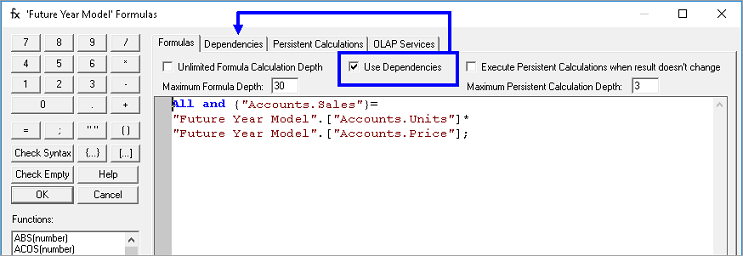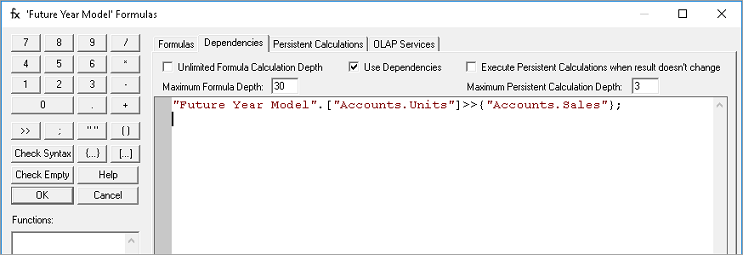Dependencies EngineThis model is the optimization of the Formula Engine model. The Dependencies Engine allows the user, basing on a certain formula in the Cube, to write dependencies to tell the engine what values it needs to calculate. For full description of the Dependency feature refer to the Dependencies part of the Formulas section, as this is simply a description of the engine.

It is important to know that dependencies values must be written correctly and it must be valid before it could work. However, the improvements that may be seen could easily be several orders of magnitude based on the complexity of your model and how many detail points one is trying to calculate and roll into aggregate. It is imperative to know that dependencies can only be written on Detail level formulas. If a formula can be written and performed at the Aggregate level or the ‘ALL’ level, then there is no need to write a dependency formula for that particular Cube.

In this example we have a formula in the Cube that computes for Sales value by multiplying Units by Price. We are going to tell the engine to consider only Detail members that has a value for Members Units and Price.

1.   In the PowerOLAP ribbon, select Model tab and Cubes command.
The Cubes dialog box is opened.

2.   Select a Cube (in the example the Future Year Model cube was selected)
then click on Formulas button to access the Formula Editor dialog.

Upon viewing the Formulas tab it contains a formula that says Sales= Units x Price.

3.   Click on the Use Dependencies check box.4.   Select the Dependencies tab and write a dependency formula as in the example:The formula written above tells the engine to consider only calculating this formula when there is a value for Units.

5.   Check for Syntax errors by clicking on the Check Syntax button.